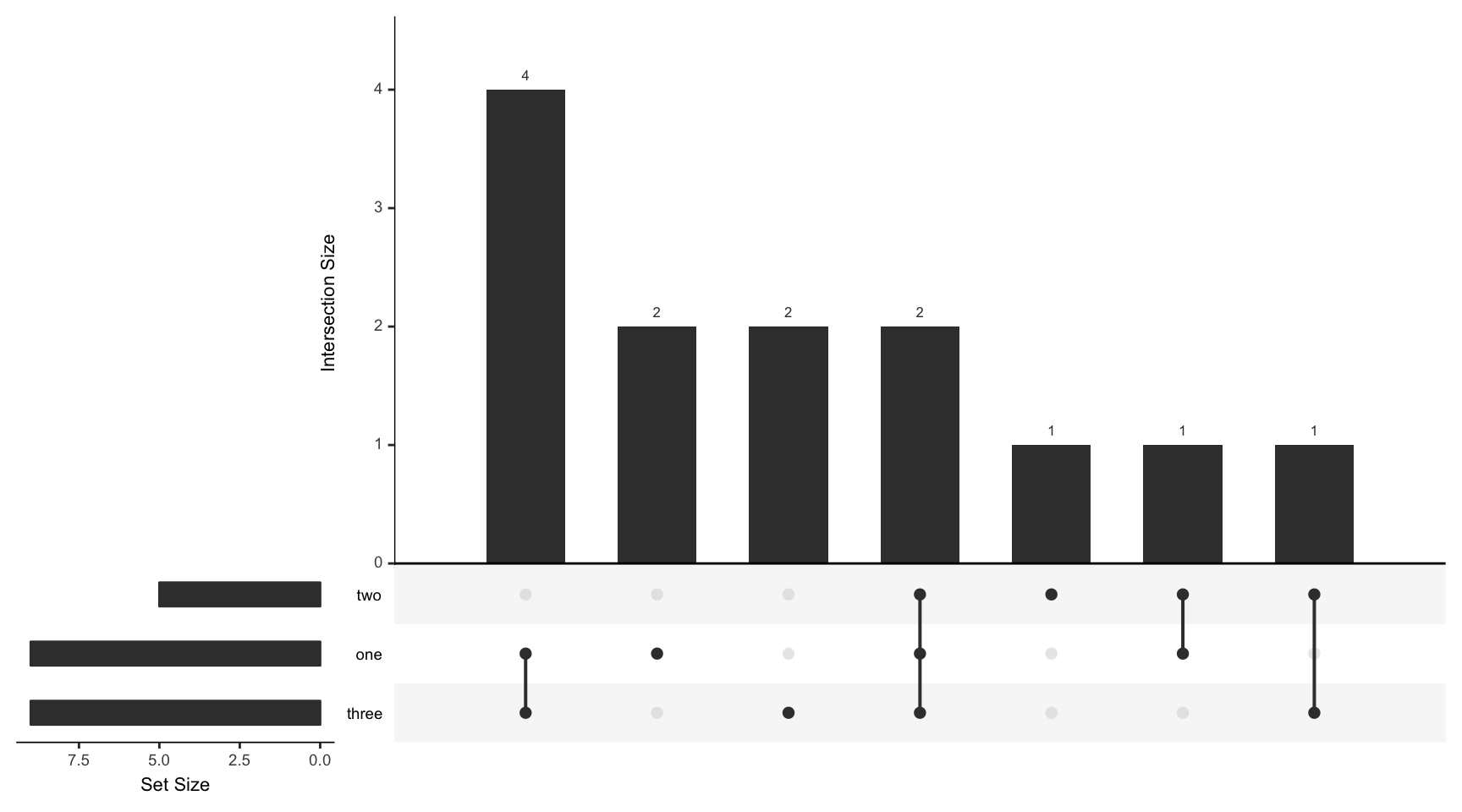For all examples the movies data set contained in the package will be used.

library(UpSetR)
movies <- read.csv(system.file("extdata", "movies.csv", package = "UpSetR"),
header = T, sep = ";")

Example 1: Alternative Input Formats

Before we start producing examples using the movies dataset, it is important to know alternative formats to input data. In some cases, the data you have may not be in the form of a file. In UpSetR there are two built in converter functions fromList and fromExpression that take alternative data formats. The fromList function takes a list of named vectors and converts them into a data frame compatible with UpSetR. The fromExpression function takes a vector that acts as an expression. The elements of the expression vector are the names of the sets in an intersection, seperated by an amerpsand (&), and the number elements in that intersection.

# example of list input (list of named vectors)
listInput <- list(one = c(1, 2, 3, 5, 7, 8, 11, 12, 13), two = c(1, 2, 4, 5,
10), three = c(1, 5, 6, 7, 8, 9, 10, 12, 13))

# example of expression input
expressionInput <- c(one = 2, two = 1, three = 2, `one&two` = 1, `one&three` = 4,
`two&three` = 1, `one&two&three` = 2)

Note that both of these inputs contain the same data. To generate an UpSet plot with these inputs set the data paramter equal to either fromList(listInput) or fromExpression(expressionInput).

upset(fromList(listInput), order.by = "freq")upset(fromExpression(expressionInput), order.by = "freq")Example 2: Choosing the Top Largest Sets and Plot Formatting

When not specifying specific sets, nsets selects the n largest sets from the data. number.angles determines the angle (in degrees) of the numbers above the intersection size bars. point.size changes the size of the circles in the matrix. line.size changes the size of the lines connecting the circles in the matrix. mainbar.y.label and sets.x.label can be used to change the axis labels on the intersection size bar plot and set size bar plot, respectively. Recently added, text.scale allows scaling of all axis titles, tick labels, and numbers above the intersection size bars. text.scale can either take a universal scale in the form of an integer, or a vector of specific scales in the format: c(intersection size title, intersection size tick labels, set size title, set size tick labels, set names, numbers above bars).

upset(movies, nsets = 6, number.angles = 30, point.size = 3.5, line.size = 2,
mainbar.y.label = "Genre Intersections", sets.x.label = "Movies Per Genre",
text.scale = c(1.3, 1.3, 1, 1, 2, 0.75))